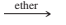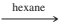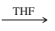×
Get Full Access to Organic Chemistry - 8 Edition - Chapter 10 - Problem 12p
Get Full Access to Organic Chemistry - 8 Edition - Chapter 10 - Problem 12p

×

# Predict the products of the following | Ch 10 - 12PISBN: 9780321768414 33

## Solution for problem 12P Chapter 10

Organic Chemistry | 8th Edition

• Textbook Solutions
• 2901 Step-by-step solutions solved by professors and subject experts
• Get 24/7 help from StudySoup virtual teaching assistantsOrganic Chemistry | 8th Edition

4 5 1 243 Reviews
14
0
Problem 12P

Predict the products of the following reactions.(a)  $$\mathrm {CH_3CH_2Br}$$  +  $$\mathrm {Mg}$$(b)  isobutyl iodide  +  $$2\ \mathrm{Li}$$(c)  1-bromo-4-fluorocyclohexane  +  $$\mathrm {Mg}$$(d)  $$\mathrm {CH_2=CCl-CH_2CH_3}$$ +  $$2\ \mathrm{Li}$$

Equation Transcription:Text Transcription:

CH_{3}CH_{2}Br

Mg

2 Li

Mg

THF

CH_{2}=CCl-CH_{2}CH_{3}

2 Li

Step-by-Step Solution:
Step 1 of 4

Solution 12P:

Here, we are going to predict the products of the following reactions.

Step1:

Organometallic compounds are those which are having Lithium(Li) and magnesium (Mg) metal with alkynyl group. It would be either organolithium reagent or organomagnesium compound(Grignard reagent).

(a)

Organometallic compounds having Li and Mg are frequently used for the synthesis of alcohols.

It has been synthesized by the following way:The synthesized compound is called Grignard reagent.

Step 2 of 4

Step 3 of 4

##### ISBN: 9780321768414

This textbook survival guide was created for the textbook: Organic Chemistry, edition: 8. Organic Chemistry was written by and is associated to the ISBN: 9780321768414. This full solution covers the following key subjects: predict, products, reactions. This expansive textbook survival guide covers 25 chapters, and 1336 solutions. The full step-by-step solution to problem: 12P from chapter: 10 was answered by , our top Chemistry solution expert on 05/06/17, 06:41PM. The answer to “?Predict the products of the following reactions. (a) $$\mathrm {CH_3CH_2Br}$$ + $$\mathrm {Mg}$$ (b) isobutyl iodide + $$2\ \mathrm{Li}$$ (c) 1-bromo-4-fluorocyclohexane + $$\mathrm {Mg}$$ (d) $$\mathrm {CH_2=CCl-CH_2CH_3}$$ + $$2\ \mathrm{Li}$$ Equation Transcription:Text Transcription:CH_{3}CH_{2}BrMg2 LiMgTHFCH_{2}=CCl-CH_{2}CH_{3}2 Li” is broken down into a number of easy to follow steps, and 35 words. Since the solution to 12P from 10 chapter was answered, more than 391 students have viewed the full step-by-step answer.

## Discover and learn what students are asking

Calculus: Early Transcendental Functions : Basic Differentiation Rules and Rates of Change
?Finding a Value In Exercises 65–70, find k such that the line is tangent to the graph of the function. Function

Unlock Textbook Solution